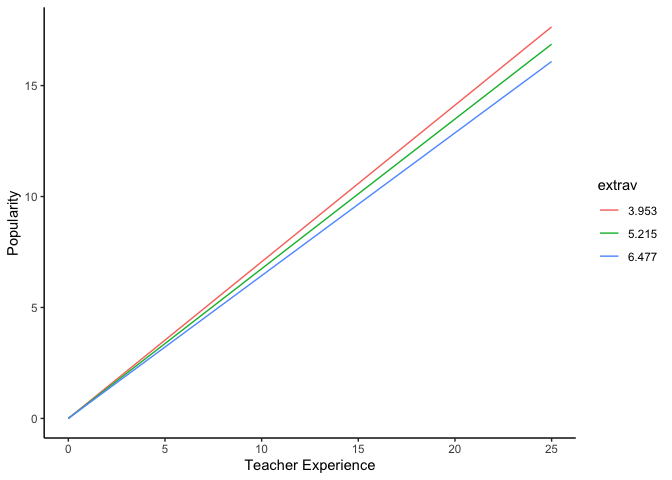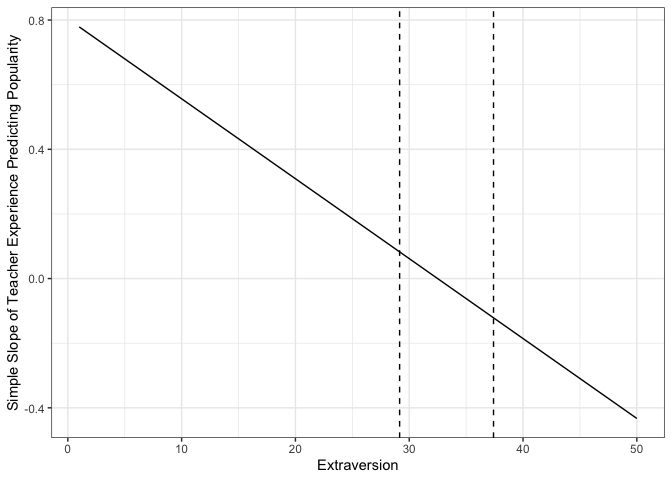# Guest Post: Interpreting Interactions in Multilevel Models

This is a guest post by Jeremy Haynes, a doctoral student at Utah State University.

# Fitting Model and Specifying Simple Intercepts and Simple Slopes

Statistical models
Model 1
(Intercept) -1.21***
(0.27)
sexgirl 1.24***
(0.04)
extrav 0.80***
(0.04)
texp 0.23***
(0.02)
extrav:texp -0.02***
(0.00)
AIC 4798.45
BIC 4848.86
Log Likelihood -2390.23
Num. obs. 2000
Num. groups: class 100
Var: class (Intercept) 0.48
Var: class extrav 0.01
Cov: class (Intercept) extrav -0.03
Var: Residual 0.55
***p < 0.001, **p < 0.01, *p < 0.05

1. Write out model equation yi**j = γ00 + γ10Xi**j + γ20Xi**j + γ01Zj + γ21Xi**jZj + μ0j + μ2j + ei**j

2. Separate fixed effects from random effects yi**j = (γ00 + γ10Xi**j + γ20Xi**j + γ01Zj + γ21Xi**jZj) + (μ0j + μ2j + ei**j)

3. Derive prediction equation E[y|X, Z] = γ̂00 + γ̂10Xi**j + γ̂20Xi**j + γ̂01Zj + γ̂21Xi**jZj

4. Separate simple intercept from simple slope - this indirectly defines the focal predictor and moderator E[y|X, Z] = (γ̂00 + γ̂10Xi**j + γ̂20Xi**j) + (γ̂01Zj + γ̂21Xi**jZj)

5. Formally define focal predictor (Level 2: Z) and moderator (Level 1: X) E[y|X, Z] = (γ̂00 + γ̂10Xi**j + γ̂20Xi**j) + (γ̂01 + γ̂21Xi**j)Zj

6. Define simple intercept (ω0) and simple slope (ω1) ω0 = γ̂00 + γ̂10Xi**j + γ̂20Xi**j

ω1 = γ̂01 + γ̂21Xi**j

# Methods of Probing Interactions (Preacher et al., 2006)

## Simple Slopes Technique

1. Specify conditional values of X (EXT) to evaluate significance for simple slope of y regressed on Z (TEXP)
• For continuous variables, this requires the “pick-a-point” method (Rogosa, 1980)
• First, I get some descriptives and will use the mean, +1 SD, and -1 SD (Cohen & Cohen, 1983)
• Values of 3.953, 5.215, and 6.477 for X

ω1 = γ̂01 + γ̂21Xi**j

 Statistic N Mean St. Dev. Min Pctl(25) Pctl(75) Max extrav 2,000 5.215 1.262 1 4 6 10
1. Calculate standard error of ω̂1
• Variance of ω̂1
 var(ω̂1 z) = var(γ̂01) + 2Xcov*(*γ̂*01, *γ̂*21) + *X*2*va**r(γ̂21)
• Covariance matrix
## 5 x 5 Matrix of class "dpoMatrix"
##               (Intercept)       sexgirl        extrav          texp   extrav:texp
## (Intercept)  7.392993e-02  1.764158e-05 -9.462046e-03 -4.187639e-03  5.370955e-04
## sexgirl      1.764158e-05  1.312796e-03 -9.458320e-05 -2.852607e-05  3.075874e-06
## extrav      -9.462046e-03 -9.458320e-05  1.609389e-03  5.400536e-04 -9.232641e-05
## texp        -4.187639e-03 -2.852607e-05  5.400536e-04  2.824846e-04 -3.686219e-05
## extrav:texp  5.370955e-04  3.075874e-06 -9.232641e-05 -3.686219e-05  6.526482e-06

• Calculate SE of ω̂1 for each value of X
1. Form critical ratios for each value of X
$t = \\frac{\\hat\\omega\_1 - 0}{SE\_{\\hat\\omega\_1}}$
1. Calculate d**f and critical t-values
• Degrees of freedom = N − p − 1

• Critical t-values

1. Determine significance
• If any of the t-values are above or below  ± t-crit, respectively, then the simple slope of the focal predictor (Z) is significant at that conditional value of X
##  "t-crit: -1.96116040314397" "t-crit: 1.96116040314397"
##  "t-value for lower conditional value: 22.5300221770147"
##  "t-value for middle conditional value: 23.5026768695656"
##  "t-value for upper conditional value: 24.5447253519584"

1. Plot that S**t
• Calculating predicted values
• PlotConclusion: The slope of teacher experience is significantly different from 0 at 1 SD of the mean and at the mean of extraversion.

## Johnson-Neyman Technique

$\\pm t = \\frac{\\hat\\omega\_1}{SE\_{\\hat\\omega\_1}}$

Expand

$\\pm t = \\frac{(\\hat\\gamma\_{01} + \\hat\\gamma\_{21}X)}{var(\\hat\\gamma\_{01}) + 2Xcov(\\hat\\gamma\_{01}, \\hat\\gamma\_{21}) + X^2 var(\\hat\\gamma\_{21})}$
1. Find values of X that solve for t
• X can be found by solving the following quadratic (Carden, Holtzman, Strube, 2017):
$X\_{lower}, X\_{upper} = \\frac{-b \\pm \\sqrt{b^2 - 4ac}}{2a}$

where

a = tα/22Var(γ̂21) − γ̂32,

b = 2tα/22Cov(γ̂1, γ̂21) − 2γ̂1γ̂21,

c = tα/22Var(γ̂1) − γ̂12

The regions of significance for the slope of teacher experience, conditioned on extraversion are significant beyond 29.1564177269881 and 37.4077675641277 points of extraversion. This is outside the range of extraversion; therefore, the slope of teacher experience is significantly different from 0 at all values of extraversion.

1. Plotting
• Calculating estimates of the slope for teacher experience as a function of extraversion